## CodeNewbie Community 🌱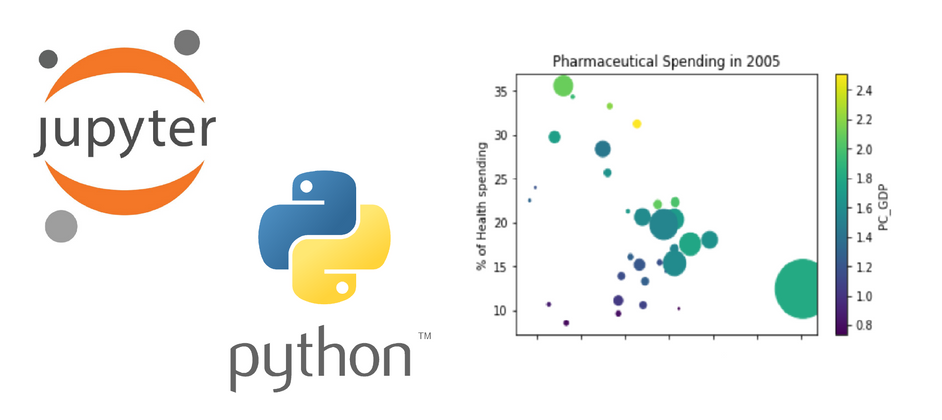# Data Analysis in Python using Jupyter Notebook - Part 3

In this tutorial we will learn how to use scatter plots and customize our graphs. We will also be making use of a new data set. In the last two articles, we looked at population over time. The population data set only included population as a measurable value and for scatter plots, at least two varying values are needed.

## Preliminary Steps

First we’ll need to import the needed packages necessary for data analysis. Then we will obtain the pharmaceutical drug spending data set from datahub.io and save it on our machine. Make sure to take note of where the csv file is saved.

``````import matplotlib.pyplot as plt
import pandas as pd
``````

Now we’ll read in the csv file. Denoting the index column as 0 (the first column) will make it easier to obtain data for specific countries.

``````spendData = pd.read_csv('../Downloads/pharm_data_csv.csv',
index_col = 0)
``````

To get a quick look at the statistical distributions of the data, we’ll do the following:

``````spendData.describe()
``````

Now we’re all set to conduct data analysis!

## Australia Pharmaceutical Spending

Using .loc, we can the obtain data specifically related to Australia’s spending.

``````aus = spendData.loc['AUS']
aus
``````

We'll use a scatter plot to show the correlation between % of Health Spending with Spending in US GDP per capita for Australia. These columns are denoted as `PC_HEALTHCP` AND `USD_CAP` respectively.

``````aus.plot(kind = 'scatter', x = 'USD_CAP', y = 'PC_HEALTHXP')
xlab = 'Spending in US GDP per capita'
ylab = '% of Health spending'
title = 'Australia Pharmaceutical Spending'
plt.xlabel(xlab)
plt.ylabel(ylab)
plt.title(title)
``````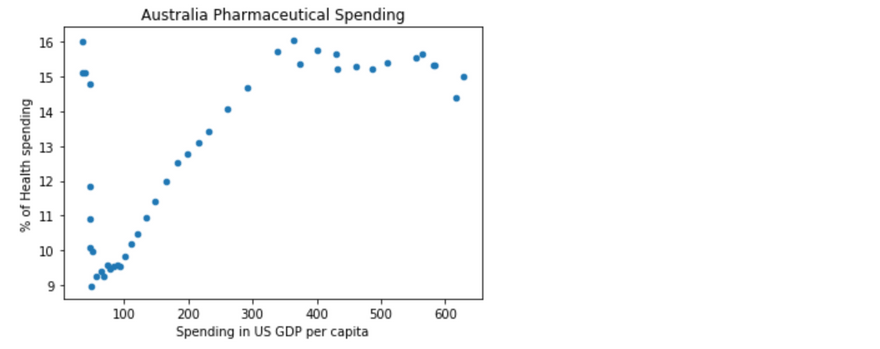This is one way of creating a scatter plot which involves using DataFrame.plot and passing in `kind = 'scatter'`. We can include a little more information by making the Total Spending denoted by the size of the data points on the graph.

``````aus.plot(kind = 'scatter', x = 'USD_CAP', y = 'PC_HEALTHXP',
s = aus['TOTAL_SPEND']/100)
xlab = 'Spending in US GDP per capita'
ylab = '% of Health spending'
title = 'Australia Pharmaceutical Spending'
plt.xlabel(xlab)
plt.ylabel(ylab)
plt.title(title)
``````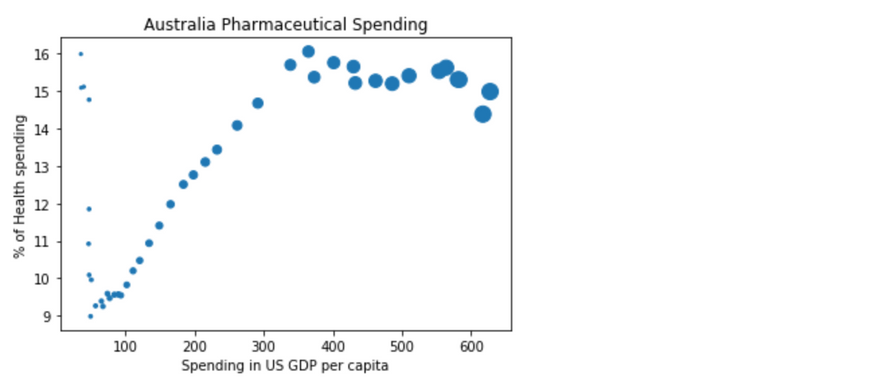Plotting the % of Heath spending against the year, we see a similar trend. The year is represented by `TIME` in the DataFrame.

``````aus.plot(kind = 'scatter', x = 'TIME', y = 'PC_HEALTHXP',
s = aus['TOTAL_SPEND']/100)
xlab = 'Year'
ylab = '% of Health spending'
title = 'Australia Pharmaceutical Spending'
plt.xlabel(xlab)
plt.ylabel(ylab)
plt.title(title)
``````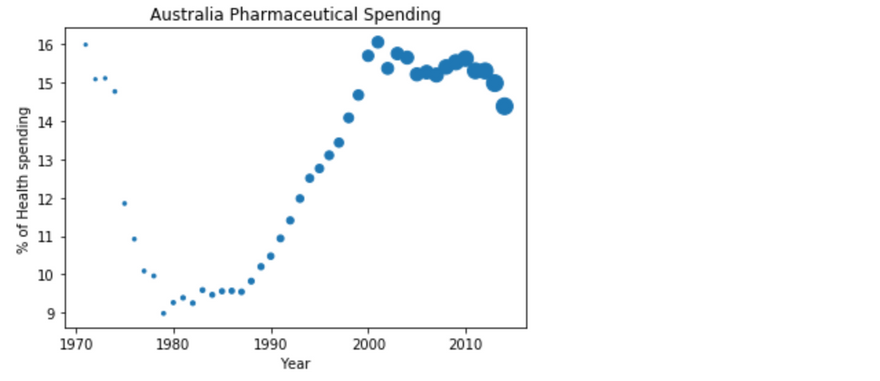## Pharmaceutical Spending in 2005

Now we’ll compare the spending of all countries in 2005. To obtain all the data, we’ll do the following:

``````spend2005 = spendData.loc[(spendData.TIME == 2005)]
spend2005
``````

Similar to what we did with Australia, we’ll look at the correlation between % of Health Spending with Spending in US GDP per capita for all countries in 2005. The size of each point corresponds to Total Spending. This time we'll be using DataFrame.plot.scatter. Using this syntax to create the scatter plot will allow us to add a colormap later on.

``````spend2005.plot.scatter(x = 'USD_CAP', y = 'PC_HEALTHXP',
s = spend2005['TOTAL_SPEND']/100)
xlab = 'Spending in US GDP per capita'
ylab = '% of Health spending'
title = 'Pharmaceutical Spending in 2005'
plt.xlabel(xlab)
plt.ylabel(ylab)
plt.title(title)
``````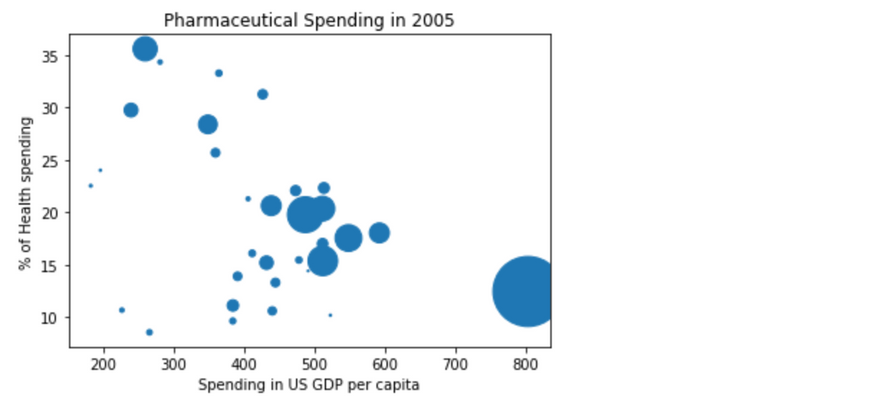The graph shows much more variation and is no longer linear. To go a step further, we can add another piece of data from the table, the percentage of GDP denoted as `PC_GDP`.

``````spend2005.plot.scatter('USD_CAP', 'PC_HEALTHXP',
s = spend2005['TOTAL_SPEND']/100, c = 'PC_GDP',
colormap = 'viridis')
xlab = 'Spending in US GDP per capita'
ylab = '% of Health spending'
title = 'Pharmaceutical Spending in 2005'
plt.xlabel(xlab)
plt.ylabel(ylab)
plt.title(title)
``````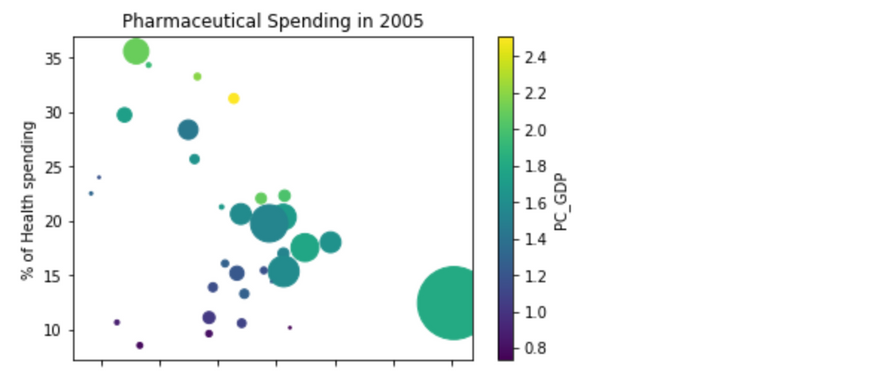This graph adds a colormap to denote the percentage of GDP. We get a good view of how all these values appear compared against each other.

The expected code execution is located here for reference.

## Closing

In this tutorial we learned to create scatter plots, closing out the series. We’ve conducted basic data analysis on countries around the world and looked at both the population trends and pharmaceutical drug spending. Congrats on making it to the end of the series, you've learned a lot!